• 2021-05-15 16:19:30

该楼层疑似违规已被系统折叠 隐藏此楼查看此楼

public static void main(String[] args) throws InterruptedException{

long starttime,endtime;

int[] num=new int;

for(int i=1;i<=20000;i++){

num[i]=(int) (Math.random()*1000000);

}

starttime=System.currentTimeMillis();

sort1.start();

sort2.start();

sort1.join();

sort2.join();

endtime=System.currentTimeMillis();

//BubbleSort(num);

for(int j=1;j<=20000;j++){

System.out.println( "Value: " + num[j]);

}

public void run(int[] num){

int temp;

for(long k=start;k<=end;k+=2){

for(int i=0 ; i < num.length ; ++i){

for(int j=0; j

if(num[j] > num[j + 1]){

temp = num[j];

num[j] = num[j + 1];

num[j + 1] = temp;

}

}

}

}

}

更多相关内容
• java课的课后作业，要求实现数组的多线程冒泡排序并实现可视化，自己写了一个奇偶排序的多线程，但效率较低，现在回顾一下老师课上讲的程序。 生成的界面如上 首先实现Button1的功能，生成随机的指定大小的数组 ...

java课的课后作业，要求实现数组的多线程冒泡排序并实现可视化，自己写了一个奇偶排序的多线程，但效率较低，现在回顾一下老师课上讲的程序。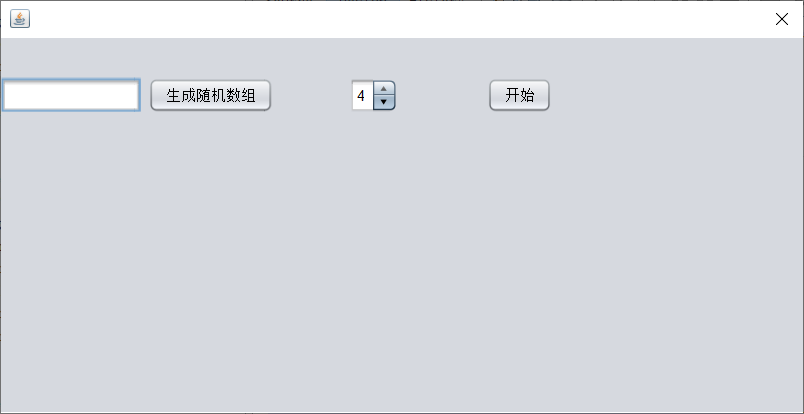生成的界面如上
首先实现Button1的功能，生成随机的指定大小的数组

private void jButton1ActionPerformed(java.awt.event.ActionEvent evt) {
long len=(Long)jFormattedTextField1.getValue();
int arr[]=new int[(int)len];
for(int i=0;i<len;i++)
{
arr[i]=(int)(Math.random()*10000);
}
SortPane.ar=arr;

this.repaint();
}


接着实现选择线程的按钮

    private void jButton2ActionPerformed(java.awt.event.ActionEvent evt) {

Object value=jSpinner1.getValue();
int tn= Integer.parseInt(value.toString());
SortPane.mode=new int[tn];
BubbleOrder[]bo=new BubbleOrder[tn];

for(int i=0;i<tn;i++)
{
bo[i]= new BubbleOrder((SortPane)jPanel1,i,tn,SortPane.ar);

}

try {
for(int i=0;i<tn;i++)
{
bo[i].start();

}
}


输入的值为tn，最后生成tn个线程
在SortPane中实现用直线表示数字大小的方法为：

    public void paint(Graphics g) {
if(ar==null)
super.paint(g); //To change body of generated methods, choose Tools | Templates.
else{
g.setColor(Color.gray);

float w=this.getSize().width;
float h=this.getSize().height;
g.fillRect(0, 0, this.getSize().width, this.getSize().height);
g.setColor(Color.red);
float w1=w/ar.length;
w1=w1<1?1:(int)(w1+0.5);
for(int i=0;i<ar.length;i++){

g.drawLine((int)(i*w1), (int)h,(int)(i*w1) ,(int) (h*(1-ar[i]/10000.0)));//drawLine中的4个参数表示两个点的坐标
}

}

}


最重要的是如何实现多线程的冒泡排序以及使各线程之间同步，假设项目中使用的4个线程的冒泡排序，那么第一个线程排序下标为0,4,8…的数，第二个线程排序下标为1,5,9…的数，由此类推，当四个线程均排到最后一个数时再对最后四个数字进行排序从而找到整个数组中最小的数，找到最小的数之后再对剩下的数组按照相同的方法排序，直到只剩下4个数时用任意一个线程去排序即可得到有序的数组，下面对代码的每一步进行分析。
1.将主函数中的参数传到各线程中，对于各参数的意义放到后面分析。

 public BubbleOrder(SortPane sp, int id, int tn, int ar[]) {
this.id = id;
this.tn = tn;
ot = (int) Math.pow(tn, 2) - 1;
this.ar = ar;
this.sp = sp;
BubbleOrder.len = ar.length;

}


2.对其进行数组每次排序后对最后四个数排序和对剩下的最后四个数排序的函数sort1进行分析

    void sort1(int len) {
if (len > tn) {//判断是每次排序的最后找最小值还是对剩下的最后四个数
for (int k = len - tn; k < len - 1; k++) {//一层for循环找最小值
if (ar[k] < ar[k + 1]) {
int ls = ar[k];
ar[k] = ar[k + 1];
ar[k + 1] = ls;
}

}
} else {//两层for循环进行排序
for (int k = len; k > 0; k--) {
for (int j = 0; j < k - 1; j++) {
if (ar[j] < ar[j + 1]) {
int ls = ar[j];
ar[j] = ar[j + 1];
ar[j + 1] = ls;
}
}
}
exit = true;
}
}


3.再对其中的排序函数进行分析，很容易知道此处的tn为线程数

    void sort(int len) {
if (id > len - tn) {
exit = true;//当剩余需排序的长度小于线程数时退出，然后采用单线程排序即可
}
for (int k = id; k < len - tn; k = k + tn) {//id是各线程声明的整型数值，表示线程的名字和线程排序的起点
if (ar[k] < ar[k + tn]) {//将较小的数换到后面
int ls = ar[k];
ar[k] = ar[k + tn];
ar[k + tn] = ls;
}
}
}


3.分析线程里面的run函数
一个for循环中，首先进行冒泡排序，然后用一个waiting表示当前线程已完成排序，等待其他线程，已完成排序的线程SortPane.get(id)方法得到false，在sortPane中声明的mode数组存储各线程的完成情况，若完成则赋值为1，

        public void run() {
while (!exit) {
System.out.print("Thread " + id + "   sorting\r\n");
sort(len);
//synchronized(SortPane.ar){
waiting = true;
try{
System.out.print("Thread " + id + "   waiting\r\n");
if(issorted(SortPane.mode))
{
sort1(len);
sp.repaint();
BubbleOrder.len--;
if (BubbleOrder.len==tn) {//当仅剩下和线程相等的数组时直接用sort1函数排序
sort1(len);
sp.repaint();
break;
}
SortPane.clear();
synchronized (SortPane.ii) {
SortPane.ii.notifyAll();
}
}
else
{
synchronized (SortPane.ii) {
//  SortPane.ar.notify();
if (exit) {
break;
}
SortPane.ii.wait();
}
}

}
catch (InterruptedException ex) {//好像没啥用
}



此处为SortPane的mode数组，用于表示各线程是否已完成当前线程的排序

  static   int[] mode;
{
mode[id]=1;
}


issorted为判断mode数组是否所有数字为1的函数

 boolean issorted(int a[])
{
boolean sorted=true;
for(int i=0;i<a.length;i++)
{
if(a[i]!=1) sorted=false;
}
return sorted;
}


最后的效果如下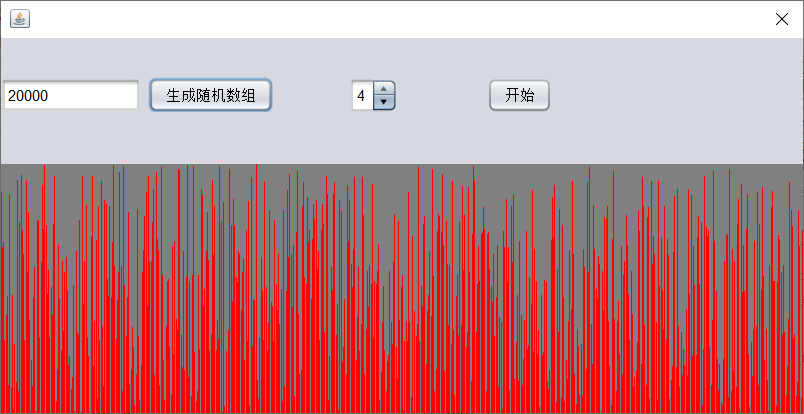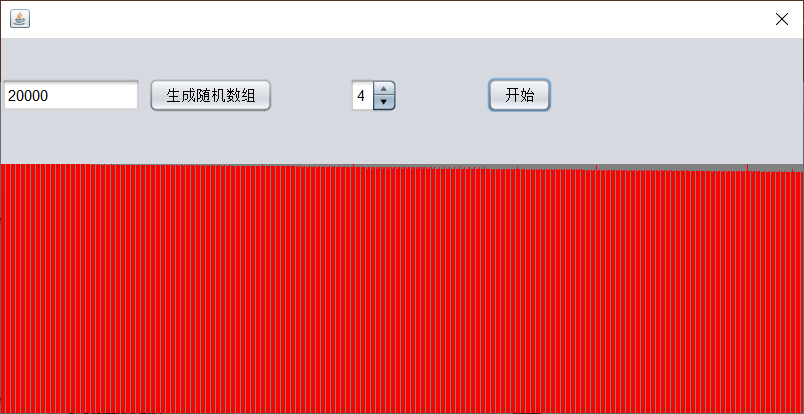展开全文• 上次传错了,这次绝对不会错了.比较适合新手哦.多线程的东西其实不要想得太复杂,自己亲手做了就会明白的
• 实验五 多线程 一、实验目的 理解Java的多线程机制。 掌握线程的创建。...题目：利用线程比较插入、冒泡排序算法的运行时间。 建立一个Person类，主要有name、age等私有成员变量，以age来对Person类的对...

## 实验五 多线程

### 一、实验目的

1. 理解Java的多线程机制。
2. 掌握线程的创建。
3. 了解线程的生命周期。
4. 了解线程的调度和优先级。
5. 掌握如何改变线程的状态。
6. 理解线程的同步机制。
7. 掌握线程的互斥锁定。
8. 掌握线程的同步运行。

### 二、实验内容和要求

题目：利用线程比较插入、冒泡排序算法的运行时间。

1. 建立一个Person类，主要有name、age等私有成员变量，以age来对Person类的对象进行排序（升序）。该类实现Comparable接口，故必须实现方法public int compareTo(Object p)。
2. 了解Comparable接口。
3. 写两个类分别实现插入、冒泡排序，并获取其运行时间。
4. 建立线程实现排序，注意一个时刻只让一个排序线程运行。
5. 在main方法里启动两个线程分别实现插入和冒泡排序。

### 三、实验代码

(1) 建立一个Person类

public class Person implements Comparable<Person>{

private String name;
private int age;

public Person(String s, int i) {
name = s;
age = i;
}

public String getName() {
return name;
}
public void setName(String name) {
this.name = name;
}

public int getAge() {
return age;
}
public void setAge(int age) {
this.age = age;
}

public int compareTo(Person p) {
/*if(this.age > p.getAge()) {
return 1;  //正整数是大于
} else if(this.age < p.getAge()) {
return -1; //负整数是小于
} else {
return 0;  //0为等于
}*/
return this.age - ((Person)p).getAge();
}

public static void main(String args[]) {

}
}


(5) 建立一个Test类在main方法中写入如下代码

public class Test {

public static void main(String[] args) {

/*		Person p1 = new Person();
Person p2 = new Person();
Person p3 = new Person();
p1.setName("小明");
p1.setAge(15);
p2.setName("小红");
p2.setAge(12);
p3.setName("大红");
p3.setAge(13);

ArrayList<Person> list=new ArrayList<Person>();

System.out.println("排序前-------------------");
for (Person p : list) {
//System.out.println(000);
System.out.println(p.getAge());
}

Collections.sort(list);

System.out.println("排序后-------------------");
for (Person p : list) {
//System.out.println(000);
System.out.println(p.getAge());
}

System.out.println("年龄最大的为"+list.get(list.size()-1).getAge());*/

Person p[]=new Person;
int i;

for(i=0;i<p.length;i++){
p[i]=new Person("平民"+i,(int)(120*Math.random()));
}

t1.start();
t2.start();

}
}


(2) 建立插入排序类，并获取运行时间

public class insertionSort {

long t1;
long t2;

public void sort(Person[] p) {

if (p.length == 0) {
throw new NullPointerException("内容为空，没有要排序的内容！");
}

t1 = System.currentTimeMillis();

int size = 1;
t1 = System.currentTimeMillis();

while (size < p.length) {
insertionSort(p, size++, p[size - 1]);
}

t2 = System.currentTimeMillis();
System.out.println("使用插入排序使用的时间是：" + (t2 - t1) + "毫秒");
System.out.println("排序结果为：");

for (int i = 0; i < p.length; i++) {
System.out.println(p[i].getName()+" "+p[i].getAge()+";");
}

}

private void insertionSort(Person[] p, int size, Person c) {

for (int i = 0; i < size; i++) {
if (c.compareTo(p[i]) < 0) {
System.out.println(p[i]);

for (int j = size; j > i; j--) {
p[j] = p[j - 1];
}

p[i] = c;

break;
}
}
}
}


(3) 建立冒泡排序类，并获取运行时间

public class bubbleSort {

long t1;
long t2;

public void sort(Person[] p) {

if (p == null) {
throw new NullPointerException("内容为空，没有要排序的内容！");
}

Person t;
t1 = System.currentTimeMillis();

for (int i = 0; i < p.length; i++) {
for (int j = 0; j < (p.length - i - 1); j++) {
if (p[j].compareTo(p[j + 1]) > 0) {
t = p[j];
p[j] = p[j + 1];
p[j + 1] = t;
}
}
}

t2 = System.currentTimeMillis();
System.out.println("使用冒泡排序所花的时间是：" + (t2 - t1) + "毫秒");
System.out.println("排序结果为：");

for (int i = 0; i < p.length; i++) {
System.out.println(p[i].getName()+" "+p[i].getAge()+";");
}
}
}


(4) 建立线程实现排序

class ThreadB extends Thread {

String s;
Object b;
Person[] c;

public ThreadB(String b, Person[] p) {
s = b;
c = p;
}

public void run() {
synchronized (c) {
if (s.equals("冒泡排序")) {
b = new bubbleSort();
((bubbleSort) b).sort(c);
} else {
b = new insertionSort();
((insertionSort) b).sort(c);
}

System.out.println();
System.out.println();
System.out.println("相隔一秒钟执行下一次的排序或者结束！");
System.out.println();
System.out.println();

try {
} catch (Exception e) {
System.out.println(e.toString());
}
}
}
}


### 四、运行结果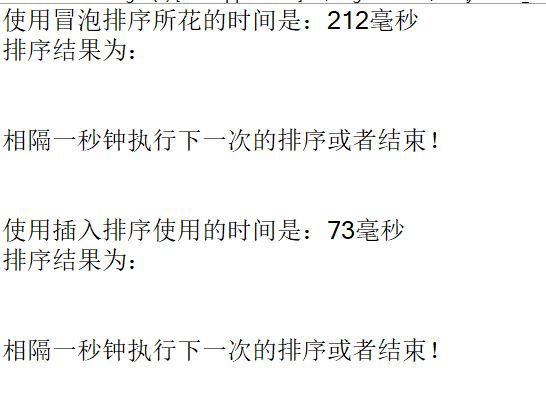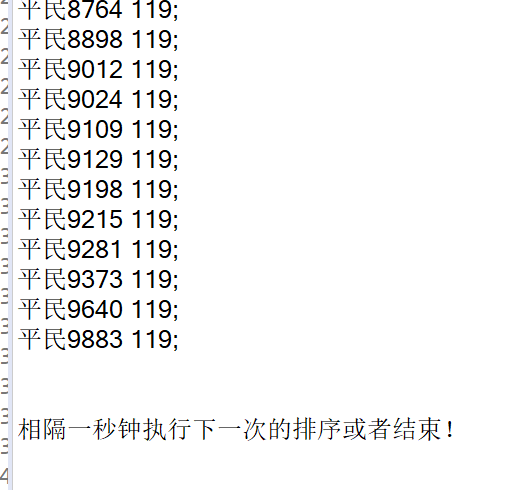展开全文• 操作系统经典实验：多线程实现排序冒泡排序与快速排序，C++编写，简单易懂
• ## 冒泡排序（c语言）

千次阅读 2021-01-11 20:37:58
冒泡排序算法详解: 1.冒泡排序简介： 冒泡排序是交换排序算法中的一个简单算法，是比较基础的。 它的特点是每一趟排序能确定一个或个关键字的最终位置。 2.冒泡排序原理介绍（假设按升序排序）： 对于给定n个...

冒泡排序算法详解:

1.冒泡排序简介： 冒泡排序是交换排序算法中的一个简单算法，是比较基础的。 它的特点是每一趟排序能确定一个或多个关键字的最终位置。
2.冒泡排序原理介绍（假设按升序排序）： 对于给定n个记录，从第一个记录开始依次对相邻的两个记录进行比较，当前面的记录大于后面的记录时，交换位置，进行一轮比较和换位后，n个记录的最大记录将位于第n位，然后对前（n-1）个记录进行第二轮比较；重复该过程，直到记录剩下一个为止。
3.举例说明：
例子1：
假设待排序序列为:6、5、4、3、2、1;
第一趟排序：5、4、3、2、1、6；
第二趟排序:4、3、2、1、5、6；
第三趟排序:3、2、1、4、5、6；
第四趟排序:2、1、3、4、5、6；
第五趟排序:1、2、3、4、5、6；
特点：
（1）一趟排序可以确定一个关键字的最终位置。
（2）共有6个元素，需要5趟排序。
例子2：
假设待排序序列为:8、12、20、10、18、24;
第一趟排序：8、12、10、18、20、24；
第二趟排序：8、10、12、18、20、24；
第三趟排序：8、10、12、18、20、24；
特点：
（1）一趟排序可以确定多个关键字的最终位置。
（2）共有6个元素，2趟排序即可完成排序。
（3）如果一趟排序没有进行关键字的位置交换，则说明排序已完成。

总结:
(1)冒泡排序中，一趟排序可以确定多个关键字的最终位置。
(2)假设有n个关键字，则冒泡排序至多进行n-1趟排序。
(3)如果一趟排序没有进行关键字的位置交换，则说明排序已完成。

4.代码实现：
代码说明:存储关键字的数组是从下标1(即a)开始存储的，下标0用作交换两个关键字的中间变量，即a
带注释的：
#include<stdio.h>
int main()
{
int a;//定义一个长度为六的数组，从下标1开始存储，a用作中间变量
int i;  //作为循环变量
printf("请输入六个整数，中间用空格隔开：\n");
//此处是从下标为1的位置开始存储这六个整数,a用作中间变量
for(i=1; i<=6; i++)
{
scanf("%d",&a[i]);
}
//下面是冒泡排序算法部分
int m= 6-1;   //m的值是待排序关键字的个数减一，即至多进行排序的趟数
int flag=1;   //flag用来判断某一趟排序中是否进行了关键字的位置交换
while(m>0 && flag==1)
{
flag = 0; //先把flag设为0，如果上一趟排序中没有进行关键字的交换，则说明排序完成，终止循环。
for(i=1; i<=m; i++)//完成一次for循环，意味着完成了一趟排序
{
if(a[i] > a[i+1])
{
a = a[i];
a[i] = a[i+1];
a[i+1] = a;
flag = 1;//如果此趟排序进行了关键字的交换，则把flag设为1，继续进行下一趟循环
}
}
m--;//完成一趟排序后，最后一个元素的下标减一
}
//排序完成输出数组
printf("冒泡排序后的结果为：");
for(i=1; i<=6; i++)
{
printf("%d ",a[i]);
}

}

展开全文算法
• 高分悬赏：Java语言利用多线程实现冒泡排序，要求和之前的快速排序一样，注意边界条件的拆分开发语言
• 1.冒泡排序的原理 每次轮回之后，未排序的值中最大的值（递增排序）都会“冒”到正确的位置上。 2. 冒泡排序的MATLAB实现 bubble.m function y=Bubble(x) % %冒泡算法： x_len=length(x); %度量数量长度，为排序做...
• VC++多线程实现三种排序算法比较----冒泡排序、快速排序、归并排序，很有意思，可以下载看看！
• 一般的，我们知道快排是优于冒泡排序的，下面就让我们用多线程验证一下，具体操作：先产生一个随机整数n（大于10），再产生n个随机正数存放于数组中，然后创建两个线程并发地对锁生成的随机整数进行排序，其中一个...快速排序 并发
• 并行的奇偶冒泡排序的实现，以及串行并行运行时间的比较。
• 实践 - 冒泡及轻者上浮 Python自带有标准GUI-图形用户界面工具包Tkinter。但Tkinter的功能相对比较简单，界面也不够漂亮，对于规模大一点的GUI应用略显不足。在当前的Python生态圈，如果读者需要一python pyqt
• 介绍了JAVA实现多线程的两种方法实例分享，有需要的朋友可以参考一下
• 说明本节主要结合排序的实例来演示多线程执行任务的流程，主要使用了线程池 ExecutorService , 闭锁 Futrue, 完成服务 CompletionService 以及最常见的冒泡排序算法基本介绍ExecutorService 线程池private static ...
•算法 c++
• 首先说明奇偶排序： 算法的思路是先排奇数序号的相邻2个，或者偶数序号的相邻两个，然后一直到序列有序为止，如下代码： #include<stdio.h> #include<stdlib.h> #include<stdbool.h> #include ...c++ cuda
• 操作系统实验，通过多线程实现 冒泡排序和快速排序算法，直观的现实每种排序的过程
• 多线程快排 #%% import threading import numpy as np #%% def partrition(l,r,A:list): pivot=A[l] while l<r: while l<r and A[r]>=pivot:r-=1 A[l]=A[r] while l<r and A[l]<=pivot :l+=1 ...python
• 1. 将插入、冒泡排序算法设计成线程，启动两个以上不同的线程同时运行，计算不同排序 的运行时间。 src/com/temp/RimingTimeOfAlgorithm.java package com.temp; import java.util.Random; /** * @Auth..java
• 易语言QQ群成员活跃度统计源码 多线程统计活跃度，统计后可以进行冒泡排序
• 题目要求编写一个C语言程序,实现基本的冒泡排序算法.算法冒泡排序,用一句话来总结:一组数中,相邻的两个数进行比较、交换,将最大(小)数交换至尾(首)部,即完成了一次冒泡排序要想对N个数字进行排序,循环N次即可.如果真...
• 冒泡排序是非常容易理解和实现，，以从小到大排序举例： 设数组长度为N。 1．比较相邻的前后二个数据，如果前面数据大于后面的数据，就将二个数据交换。 2．这样对数组的第0个数据到N-1个数据进行一次遍历后，...
• 串行冒泡排序 //串行冒泡排序 #include <iostream> #include <ctime> using namespace std; const int Num = 10000; //排序10000个数 void BubbleSort(int* array, int size) { for (int i = 0; i <...c++ 并行计算
•java 排序 选择排序...